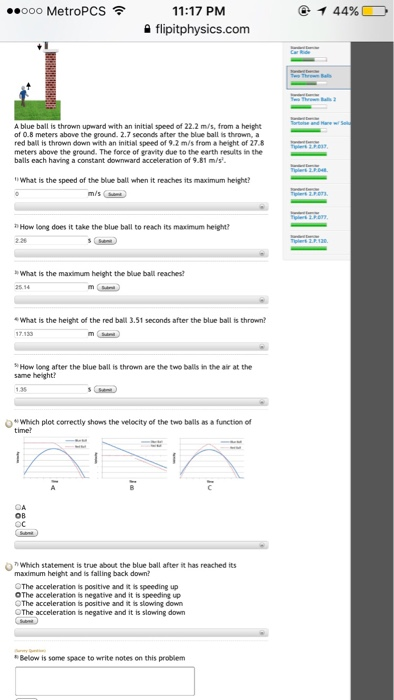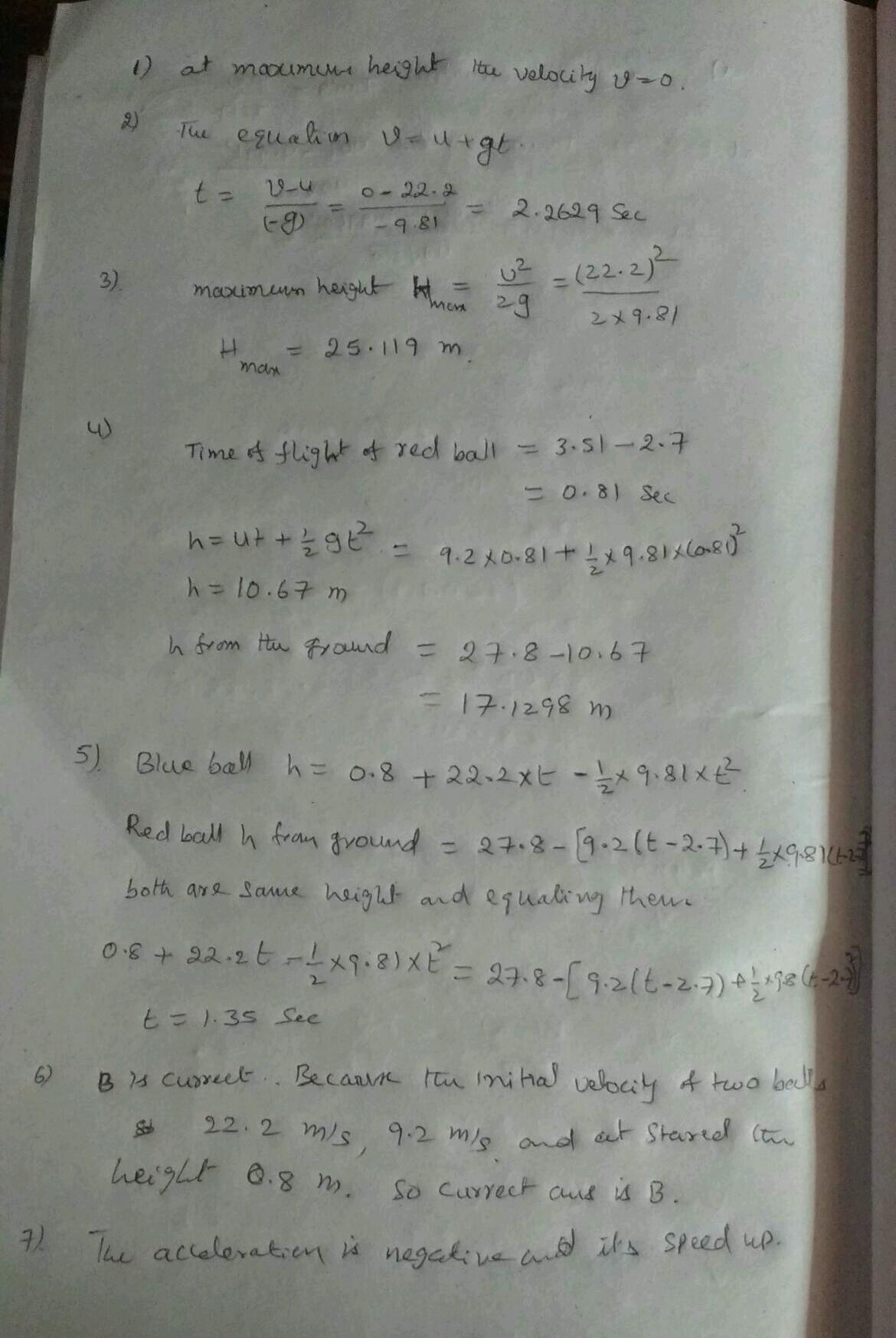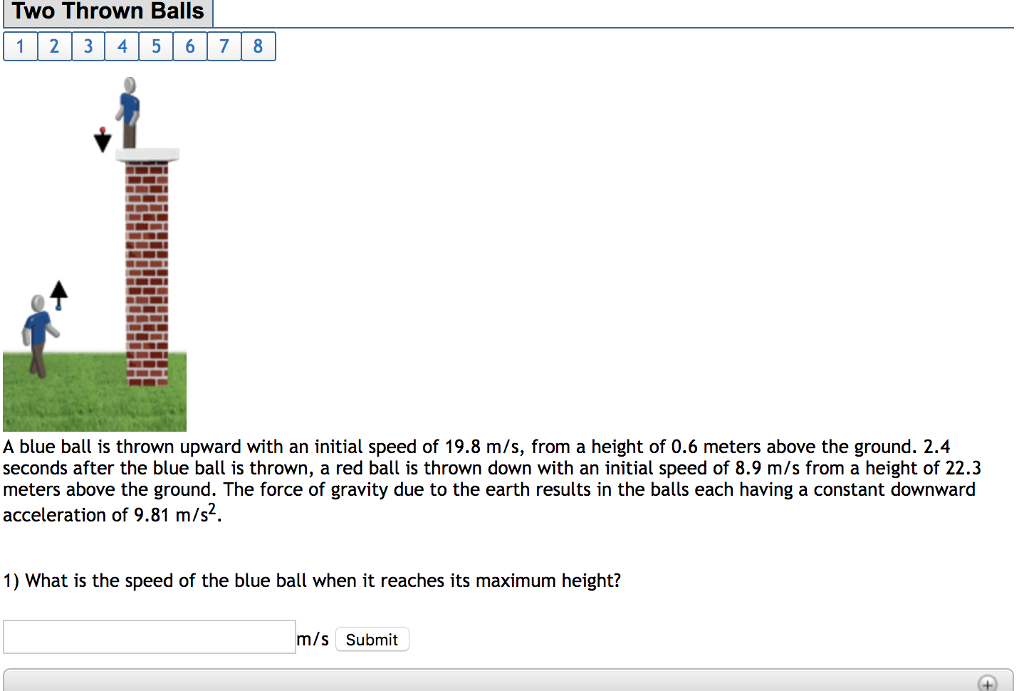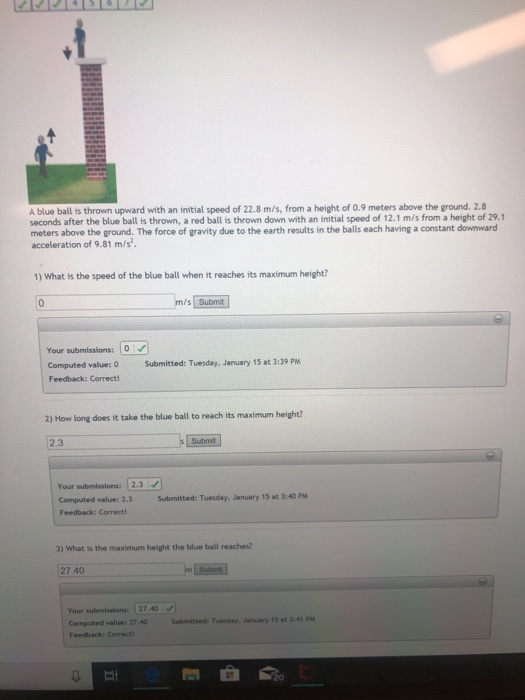Question

# Two throw A blue ball is thrown upward with an initial speed of 22.2 m/s, from...

Two throwA blue ball is thrown upward with an initial speed of 22.2 m/s, from a height of 0.8 meters above the ground. 2.7 seconds after the blue ball is thrown, a red ball is thrown down with an initial speed of 9.2 m/s from a height of 27.8 meters above the ground. The force of grandly due to the earth results in the balls each having a constant downward acceleration of 9.81 m/s^2. What is the speed of the blue ball when reaches its maximum height? m/s How long does it take the blue ball to reach its maximum height? What is the maximum height the blue ball reaches? What is the height of the red ball 3.51 seconds after the blue ball is thrown? How long after the blue ball is thrown are the two balls in the air at the same heights? Which plot correctly shows the velocity of the two balls as a function of time? Which statement is true about the blue ball after it has reached its maximum height and is falling back down? The acceleration is positive and it is speeding up. The acceleration is positive and it is speeding up. The acceleration is positive and it is slowing down. The acceleration is positive and it is speeding up. Below is some space to write notes on this problem.#### Earn Coins

Coins can be redeemed for fabulous gifts.

Similar Homework Help Questions
• ### Two Thrown Balls 1 2 3 45678 A blue ball is thrown upward with an initial...Two Thrown Balls 1 2 3 45678 A blue ball is thrown upward with an initial speed of 19.8 m/s, from a height of 0.6 meters above the ground. 2.4 seconds after the blue ball is thrown, a red ball is thrown down with an initial speed of 8.9 m/s from a height of 22.3 meters above the ground. The force of gravity due to the earth results in the bals each having a constant downward acceleration of 9.81 m/s2....

• ### A blue ball is thrown upward with an initial speed of 19.8 m/s, from a height of 0.5 meters above the ground

A blue ball is thrown upward with an initial speed of 19.8 m/s, from a height of 0.5 meters above the ground. 2.4 seconds after the blue ball is thrown, a red ball is thrown down with an initial speed of 7.9 m/s from a height of 22.5 meters above the ground. The force of gravity due to the earth results in the balls each having a constant downward acceleration of 9.81 m/s2. What is the maximum height the blue...

• ### A blue ball is thrown upward with an initial speed of 24.1 m/s, from a height...

A blue ball is thrown upward with an initial speed of 24.1 m/s, from a height of 0.8 meters above the ground. 2.9 seconds after the blue ball is thrown, a red ball is thrown down with an initial speed of 10.4 m/s from a height of 31.4 meters above the ground. The force of gravity due to the earth results in the balls each having a constant downward acceleration of 9.81 m/s2. What is the height of the red...

• ### A blue ball is thrown upward with an initial speed of 23.9 m/s, from a height...

A blue ball is thrown upward with an initial speed of 23.9 m/s, from a height of 0.5 meters above the ground. 2.9 seconds after the blue ball is thrown, a red ball is thrown down with an initial speed of 7.5 m/s from a height of 31.5 meters above the ground. The force of gravity due to the earth results in the balls each having a constant downward acceleration of 9.81 m/s^2. How long after the blue ball is...

• ### A blue ball is thrown upward with an initial speed of 20 m/s, from a height...

A blue ball is thrown upward with an initial speed of 20 m/s, from a height of 0.8 meters above the ground. 2.4 seconds after the blue ball is thrown, a red ball is thrown down with an initial speed of 5.3 m/s from a height of 22.4 meters above the ground. The force of gravity due to the earth results in the balls each having a constant downward acceleration of 9.81 m/s2. 1) How long after the blue ball...

• ### Please help with the last few parts A blue ball is thrown upward with an initial...Please help with the last few parts A blue ball is thrown upward with an initial speed of 22.8 m/s, from a height of 0.9 meters above the ground. 2.8 seconds after the blue ball is thrown, a red ball is thrown down with an initial speed of 12.1 m/s from a height of 29.1 meters above the ground. The force of gravity due to the earth results in the balls each having a constant downward acceleration of 9.81 m/s...

• ### A red ball is thrown down with an initial speed of 1.2 m/s from a height...

A red ball is thrown down with an initial speed of 1.2 m/s from a height of 25 meters above the ground. Then, 0.6 seconds after the red ball is thrown, a blue ball is thrown upward with an initial speed of 23.8 m/s, from a height of 0.8 meters above the ground. The force of gravity due to the earth results in the balls each having a constant downward acceleration of 9.81 m/s^2. 1)What is the speed of the...

• ### A red ball is thrown down with an initial speed of 1.2 m/s from a height...

A red ball is thrown down with an initial speed of 1.2 m/s from a height of 26 meters above the ground. Then, 0.4 seconds after the red ball is thrown, a blue ball is thrown upward with an initial speed of 23.8 m/s, from a height of 1 meters above the ground. The force of gravity due to the earth results in the balls each having a constant downward acceleration of 9.81 m/s2. 1) What is the speed of...

• ### A ball is thrown upwards with a speed of 19 m/s. it reaches a hight of...

A ball is thrown upwards with a speed of 19 m/s. it reaches a hight of 18.4 m above the point of release before it falls back down. Specify down as the positive direction and the initial position as the origin. If the balls position after three seconds is -12.9 meters, what is the distance traveled by the rock? The acceleration due to gravity is 9.8 m/s^2 down. The answer is 23.9 meters, how would i solve this?

• ### A ball is thrown upward from the ground with an initial speed of 16.6 m/s; at...

A ball is thrown upward from the ground with an initial speed of 16.6 m/s; at the same instant, another ball is dropped from a building 20 m high. After how long will the balls be at the same height above the ground?